# Geometry Take out Binder Pencil Do Now Sheet

• Slides: 27Geometry Take out: Binder, Pencil, Do Now Sheet, Homework Do Now: Name each figure B 1) A Agenda • Do Now/Check Homework • HW Questions • Naming, Measuring, and Drawing Angles • Angles and Protractor Practice • Ticket-to-go • HW: pg. 42 -44 #’s 1 -4, 7 -14, 34 2) F E 3) Draw a line AD with a midpoint C, then write all congruent segment using correct notation. 9/7/2010 Objective: Name, draw, label, and measure angles, and determine angle bisectorsRecap Geometrical Terms Point An exact location on a plane is called a point. Line A straight path on a plane, extending in both directions with no endpoints, is called a line. Line segment A part of a line that has two endpoints and thus has a definite length is called a line segment. Ray A line segment extended indefinitely in one direction is called a ray. 9/7/2010 Objective: Name, draw, label, and measure angles, and determine angle bisectorsAngles In Daily Life If we look around us, we will see angles everywhere. 9/7/2010 Objective: Name, draw, label, and measure angles, and determine angle bisectorsWhat Is An Angle ? When two non-collinear rays join with a common endpoint (origin) an angle is formed. Ray BA A B Common endpoint B Ray BC C Common endpoint is called the vertex of the angle. B is the vertex of ABC. Ray BA and BC are two non-collinear rays Ray BA and ray BC are called the arms of ABC. 9/7/2010 Objective: Name, draw, label, and measure angles, and determine angle bisectorsFact: We can also think of an angle formed by rotating one ray away from its initial position. 9/7/2010 Objective: Name, draw, label, and measure angles, and determine angle bisectors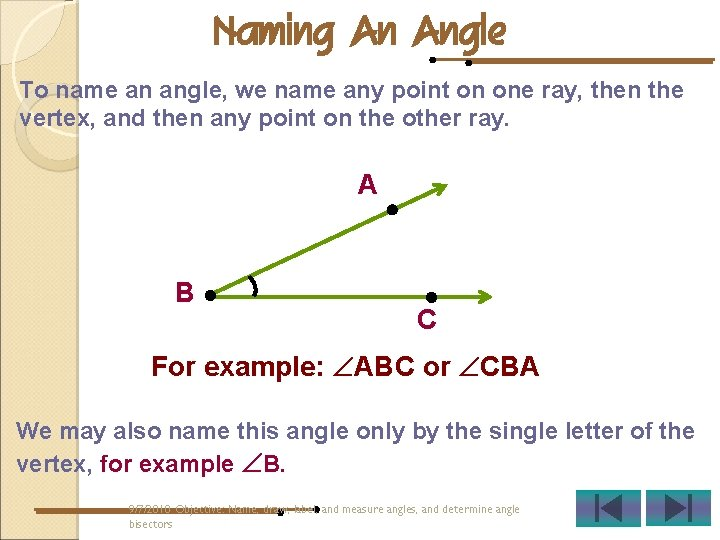Naming An Angle To name an angle, we name any point on one ray, then the vertex, and then any point on the other ray. A B C For example: ABC or CBA We may also name this angle only by the single letter of the vertex, for example B. 9/7/2010 Objective: Name, draw, label, and measure angles, and determine angle bisectorsMeasurement Of An Angle Protractor is used to measure and draw angles. Angles are accurately measured in degrees. 9/7/2010 Objective: Name, draw, label, and measure angles, and determine angle bisectorsTypes Of Angles There are four main types of angles. Right angle Acute angle A B Obtuse angle A A C B B C Straight angle A B 9/7/2010 Objective: Name, draw, label, and measure angles, and determine angle bisectors C CRight angle: An angle whose measure is 90 degrees. Straight Angle Right Angle Acute Angle 9/7/2010 Objective: Name, draw, label, and measure angles, and determine angle bisectors Obtuse AngleObtuse angle: An angle whose measure is greater than 90 degrees. Straight Angle Right Angle Acute Angle 9/7/2010 Objective: Name, draw, label, and measure angles, and determine angle bisectors Obtuse Angle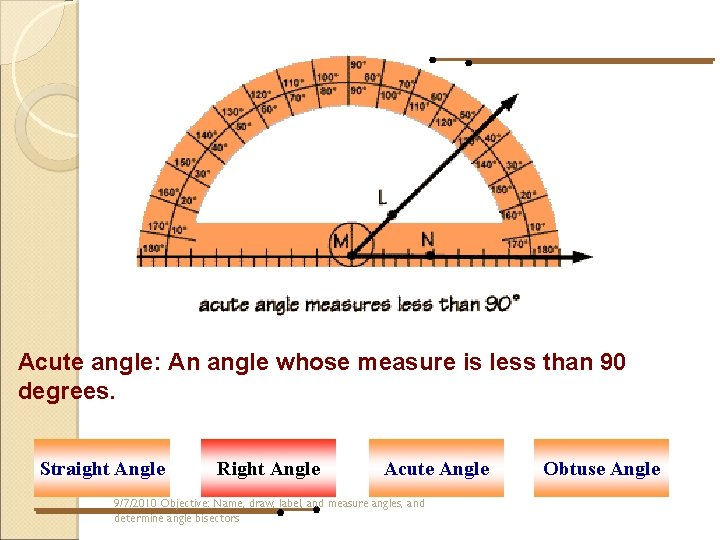Acute angle: An angle whose measure is less than 90 degrees. Straight Angle Right Angle Acute Angle 9/7/2010 Objective: Name, draw, label, and measure angles, and determine angle bisectors Obtuse AngleStraight angle: An angle whose measure is 180 degrees. Straight Angle Right Angle Acute Angle Obtuse Angle 9/7/2010 Objective: Name, draw, label, and measure angles, and determine angle bisectorsExamples Of Straight Angle 9/7/2010 Objective: Name, draw, label, and measure angles, and determine angle bisectors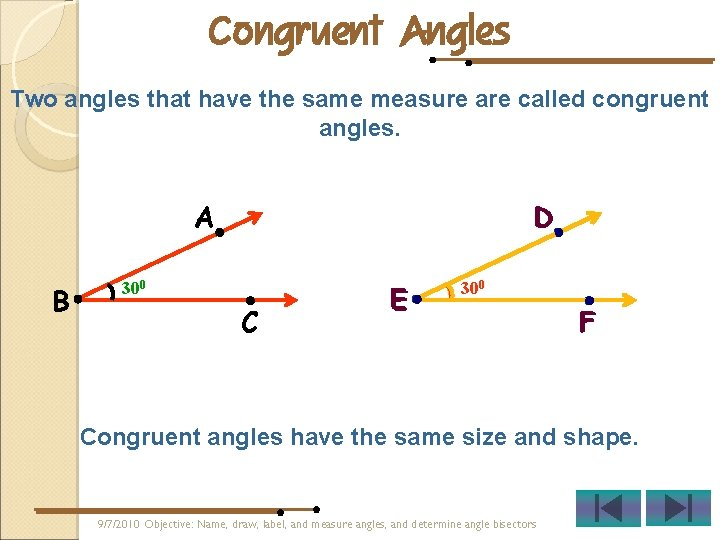Congruent Angles Two angles that have the same measure are called congruent angles. D A B 300 C E 300 F Congruent angles have the same size and shape. 9/7/2010 Objective: Name, draw, label, and measure angles, and determine angle bisectors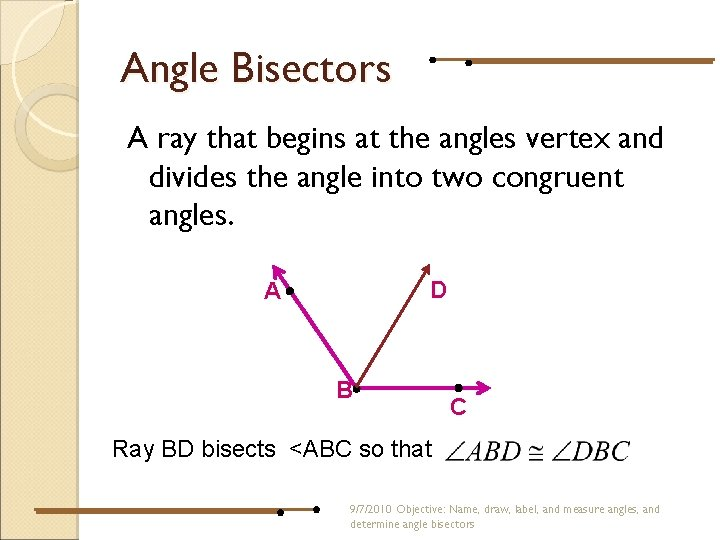Angle Bisectors A ray that begins at the angles vertex and divides the angle into two congruent angles. D A B C Ray BD bisects <ABC so that 9/7/2010 Objective: Name, draw, label, and measure angles, and determine angle bisectors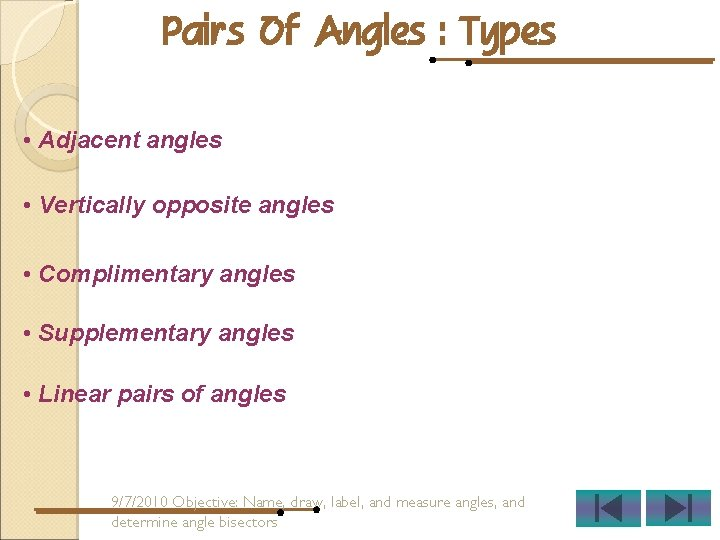Pairs Of Angles : Types • Adjacent angles • Vertically opposite angles • Complimentary angles • Supplementary angles • Linear pairs of angles 9/7/2010 Objective: Name, draw, label, and measure angles, and determine angle bisectorsAdjacent Angles Two angles that have a common vertex and a common ray are called adjacent angles. A A C D B Common ray D B Common vertex E C F Adjacent ABD and DBC ABC and Angles DEF are not adjacent angles Adjacent angles do not overlap each other. 9/7/2010 Objective: Name, draw, label, and measure angles, and determine angle bisectorsVertically Opposite Angles Vertically opposite angles are pairs of angles formed by two lines intersecting at a point. C A P B D APC = BPD APB = CPD Four angles are formed at the point of intersection. Vertically opposite angles are congruent. Point of intersection ‘P’ is the common vertex of the four angles. 9/7/2010 Objective: Name, draw, label, and measure angles, and determine angle bisectorsComplimentary Angles If the sum of two angles is 900, then they are called complimentary angles. A D 600 B E 300 C F ABC and DEF are complimentary because ABC + DEF 600 + 300 = 900 9/7/2010 Objective: Name, draw, label, and measure angles, and determine angle bisectorsContd…. If the sum of two angles is more than 900 or less than 900, then they not complimentary angles. D p 700 E F Q 300 R ÐDEF and ÐPQR are not complimentary because DEF + PQR 700 + 300 = 1000 9/7/2010 Objective: Name, draw, label, and measure angles, and determine angle bisectors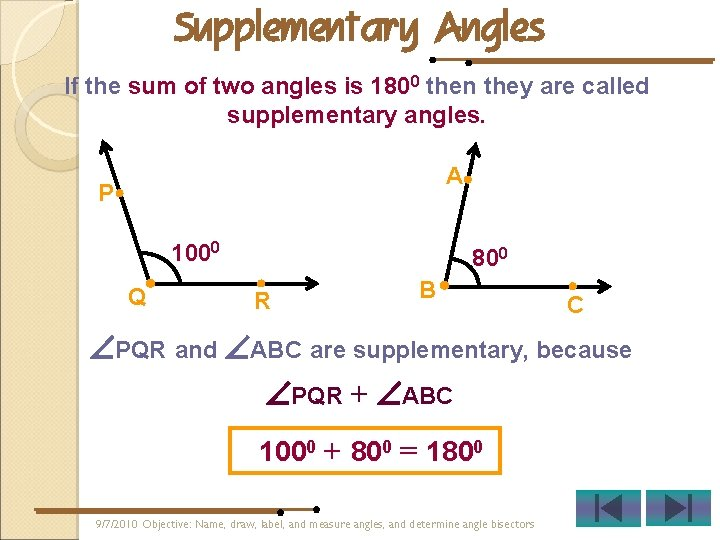Supplementary Angles If the sum of two angles is 1800 then they are called supplementary angles. A P 1000 Q 800 R B C PQR and ABC are supplementary, because PQR + ABC 1000 + 800 = 1800 9/7/2010 Objective: Name, draw, label, and measure angles, and determine angle bisectors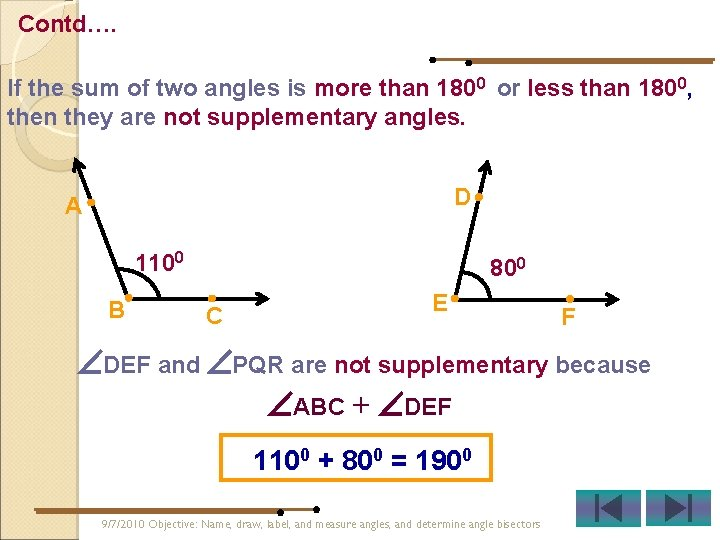Contd…. If the sum of two angles is more than 1800 or less than 1800, then they are not supplementary angles. D A 1100 B 800 C E F DEF and PQR are not supplementary because ABC + DEF 1100 + 800 = 1900 9/7/2010 Objective: Name, draw, label, and measure angles, and determine angle bisectors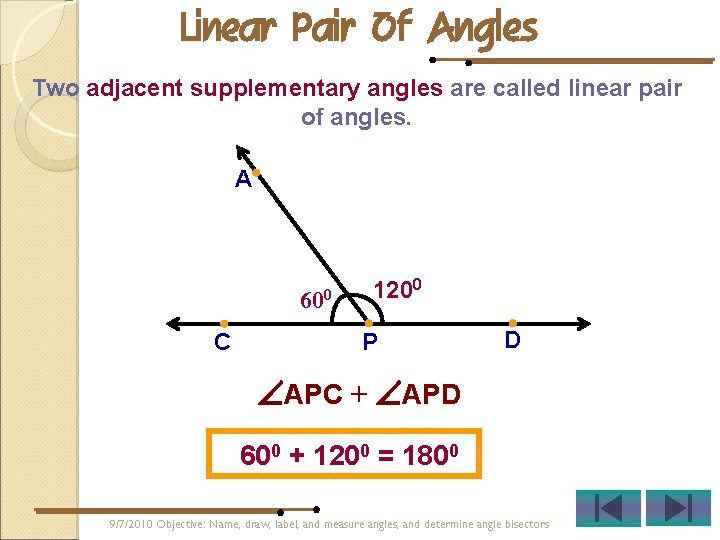Linear Pair Of Angles Two adjacent supplementary angles are called linear pair of angles. A 600 C 1200 P D APC + APD 600 + 1200 = 1800 9/7/2010 Objective: Name, draw, label, and measure angles, and determine angle bisectorsName the adjacent angles and linear pair of angles in the given figure: Adjacent angles: ABD and DBC A ABE and DBA 00 30 30 Linear pair of angles: EBA, ABC 0 0 9090 E D 0 60 600 B EBD, DBC 9/7/2010 Objective: Name, draw, label, and measure angles, and determine angle bisectors CName the vertically opposite angles and adjacent angles in the given figure: C A P B D Vertically opposite angles: APC and BPD Adjacent angles: APC and CPD APB and BPD 9/7/2010 Objective: Name, draw, label, and measure angles, and determine angle bisectors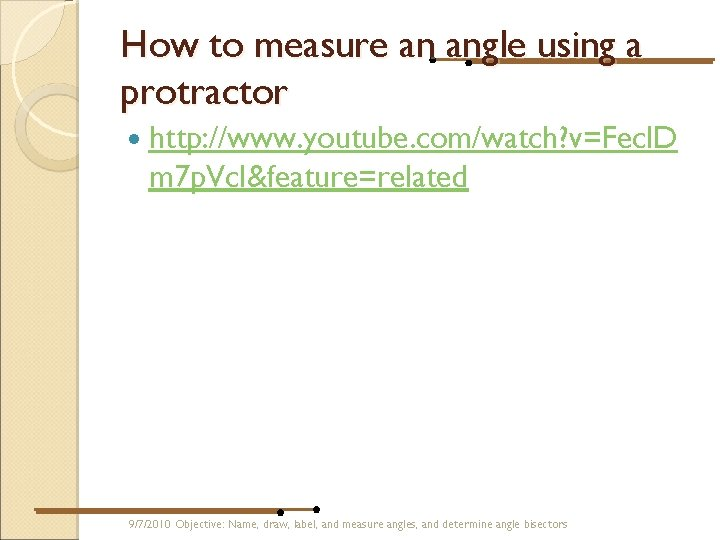How to measure an angle using a protractor http: //www. youtube. com/watch? v=Fecl. D m 7 p. Vc. I&feature=related 9/7/2010 Objective: Name, draw, label, and measure angles, and determine angle bisectors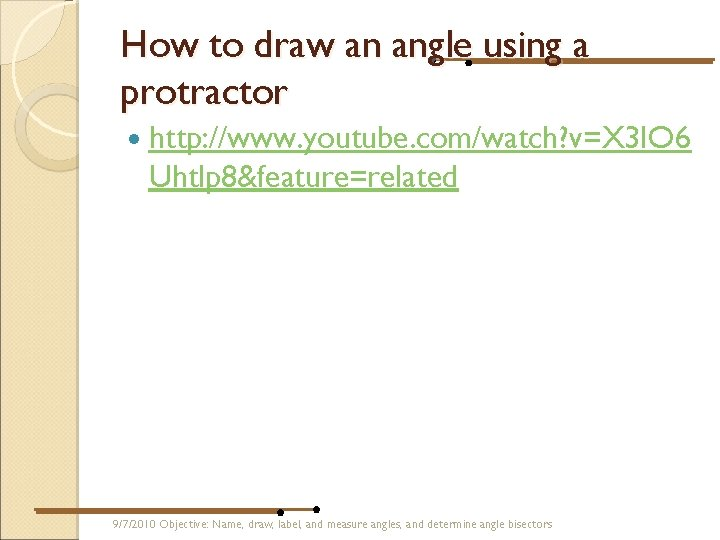How to draw an angle using a protractor http: //www. youtube. com/watch? v=X 3 IO 6 Uhtlp 8&feature=related 9/7/2010 Objective: Name, draw, label, and measure angles, and determine angle bisectors